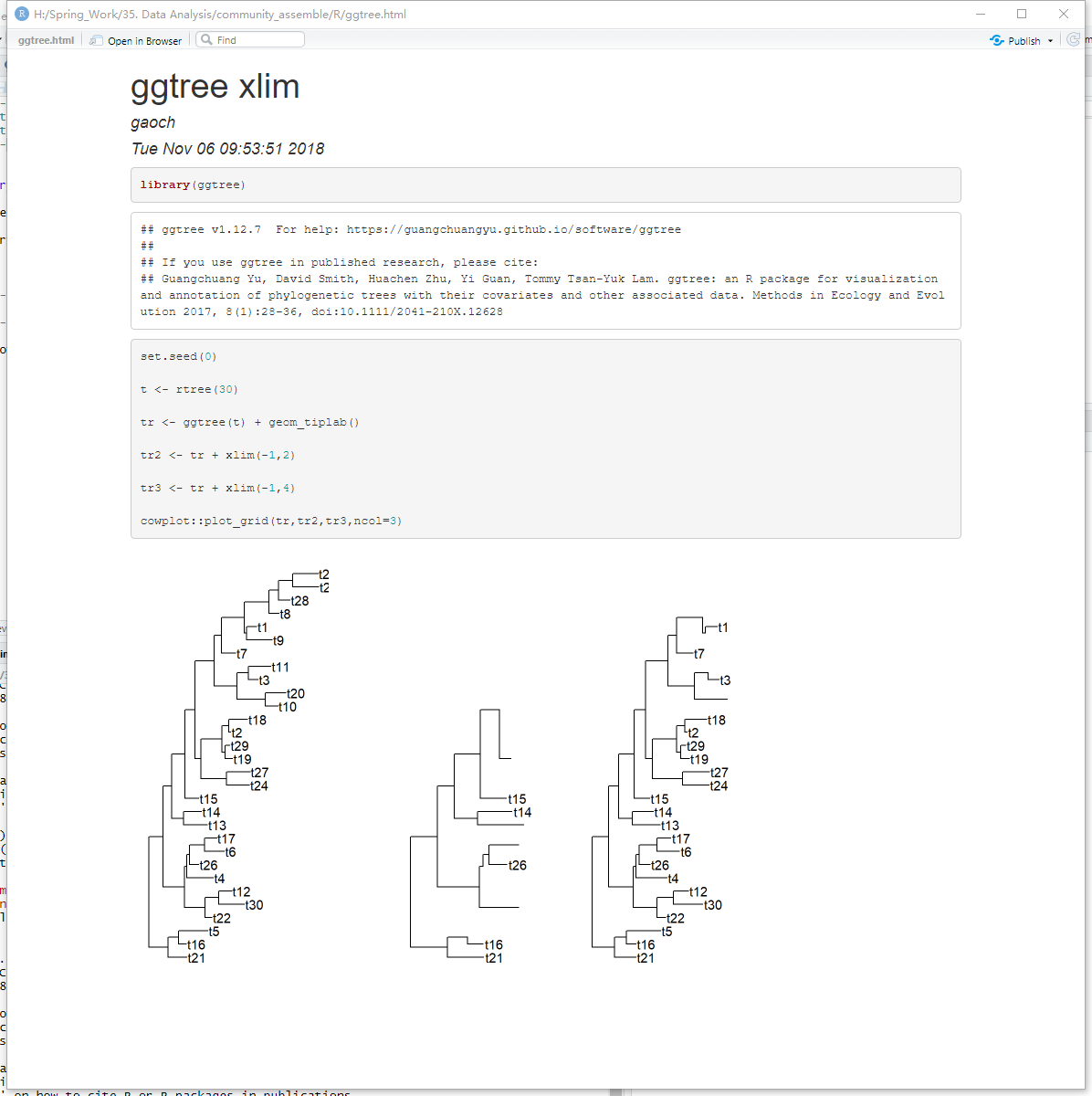# cowplot是旧爱，patchwork乃新欢

Thomas Lin Pedersen简直是个天才，最近`patchwork`动作很大，看到我都准备转投它的怀抱，把旧爱`cowplot`给扔了。

``````library(ggplot2)
p1 <- ggplot(mtcars) +
geom_point(aes(mpg, disp)) +
ggtitle('图一')

p2 <- ggplot(mtcars) +
geom_boxplot(aes(gear, disp, group = gear)) +
ggtitle('图二')

p3 <- ggplot(mtcars) +
geom_point(aes(hp, wt, colour = mpg)) +
ggtitle('图三')

p4 <- ggplot(mtcars) +
geom_bar(aes(gear)) +
facet_wrap(~cyl) +
ggtitle('图四')
``````

# 设置可视化窗口1. 你的环境有问题吧
1. 傻逼你会用吗。

# 用facet_labeller改facet_plot的panel名

I post this because I am facing the same problem: I want to change the text of the label (not the font, the face, the colour, etc: the text itself) of the leftmost panel which is automatically called “Tree” by ggtree. As far as I understand, and contrary to what is posted below, the “theme” thing of ggplot2 only allows to change the appearance of the panel header, not the text of the panel title itself… So how to go about that?

# separating legends of heatmaps in ggtree

I’ve been having great success with ggtree now and have recommended it to several colleages here in Oxford.

Is there any way to seperate the legend in heatmaps? Eg I have plotted a tree in a circular style with outer rings given extra data - I would like a seperate key for each ring - is this possible?

This is a user question. Plotting multiple heatmaps that align to the phylogenetic tree is easy with `gheatmap` function.

Here is a simple example:

``````library("ggplot2")
library("ggtree")

nwk <- system.file("extdata", "sample.nwk", package="treeio")

circ <- ggtree(tree, layout = "circular")

df <- data.frame(first=c("a", "b", "a", "c", "d", "d", "a", "b", "e", "e", "f", "c", "f"),
second= c("z", "z", "z", "z", "y", "y", "y", "y", "x", "x", "x", "a", "a"))

rownames(df) <- tree\$tip.label

p1 <- gheatmap(circ, df[, "first", drop=F], offset=.8, width=.1,
colnames_angle=90, colnames_offset_y = .25)
p2 <- gheatmap(p1, df[, "second", drop=F], offset=5, width=.1,
colnames_angle=90, colnames_offset_y = .25)``````

# pheatmap类属性：一个更好用的pheatmap

`pheatmap`我其实用的次数不多，但在帮别人解决一些问题之后，比如：

# 2018世界杯专属图层

• page 1 of 14#### Guangchuang Yu

Bioinformatics Professor @ SMU

Bioinformatics Professor

Guangzhou